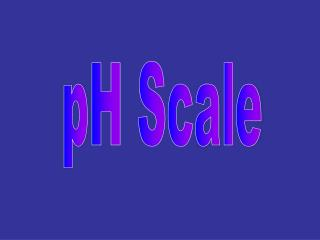Download PresentationpH scale

# pH scale - PowerPoint PPT PresentationDownload Presentation## pH scale

- - - - - - - - - - - - - - - - - - - - - - - - - - - E N D - - - - - - - - - - - - - - - - - - - - - - - - - - -
##### Presentation Transcript

1. pH scale • Logarithmic scale • expressing H+1 concentration, [H+1] • If pH changes by factor of 1, [H+1] changes by factor of 10 • pH = -log[H+1]

2. Molarity to pH To determine pH: • express [H+1] in scientific notation • remember, [ ] means concentration of whatever is inside brackets • log is the power of 10

3. Molarity to pH for Pure water there’s a tiny bit of ionization: H2O  H+1 + OH-1 • [H+1] = [OH-1] = 1 X 10-7M • pH =-log[H+1]= -(-7) = 7

4. [H+1] 1 M or 1X100 M 0.1 M or 1 X 10-1M 0.01 M or 1 X 10-2M .001 M or 1 X 10-3M pH 0 1 2 3 pH

5. pH to Molarity pH = -log[H+1], solve for [H+1] • -pH = log[H+1] • antilog(-pH) = [H+1] • say pH = 5, then –pH = -5 • antilog(-5) = 10-5 • the –pH becomes the power of 10!

6. pOH • by analogy: pOH is defined as –log[OH-1] • express [OH-1] in scientific notation • if [OH-1] = 1.0 X 10-3 M • then pOH = -log(10-3) = -(-3) = 3

7. 11 4 5 13 pH + pOH = 14 • pH = 3, pOH = • pH = 7, pOH = • pH = 10, pOH = • pH = 9, pOH = • pH = 1, pOH = 7

8. ACID or BASE? • acids: [H+1]  [OH-1] • bases: [OH-1]  [H+1]

9. pH range • 0 to 14 • with 7 being neutral • pH = 0, strongly acidic • pH = 14, strongly basic

10. antilog(-pH) = [H+1] antilog(-4) = 1 x 10-4 M 10 antilog(-10) = [OH-1] 1x10-10 M = [OH-1] if pH = 4 • [H+1] = ? • pOH = ? • [OH-1] = ?

11. 14 – 3 = 11 antilog(-11) = 1x10-11 M if the [OH-1] = 1 X 10-3 pOH = -log[OH-1] = -log(10-3) = -(-3) = 3 • pOH = ? • pH = ? • [H+1] = ?

12. 5 14 – 5 = 9 antilog(-9) = 1x10-9M if the [H+1] = 1 x 10-5 M • pH = ? • pOH = ? • [OH-] = ?

13. [H+] (M) pH [OH-] (M) pOH Acidic or Basic 3 1x10-3 1x10-11 11 A 1x10-5 5 1x10-9 9 B 1x10-2 A 1x10-12 12 2 6 B 1x10-6 8 1x10-8

14. How to safely test pH • instruments – use a pH meter • indicators – use a series of indicators • see if substance reacts with a metal other than Cu, Ag, or Au • NEVER “taste”

15. Indicator • substance that changes color over narrow pH range • use several indicators to narrow down pH range of substance